Conclusion（总结）

# 梯度下降法原理

(1) 随机设置一个初始值

(2) 计算损失函数的梯度

(3) 设置步长，步长的长短将会决定梯度下降的速度和准确度

(4) 将初值减去步长乘以梯度，更新初值，然后将这一过程不断迭代

# 使用二次函数简单实现和验证梯度下降

import numpy as np
import matplotlib.pyplot as plt

plot_x = np.linspace(-1,6,141)
plot_y = (plot_x - 2.5)**2 - 1
plt.plot(plot_x,plot_y)
plt.show()

##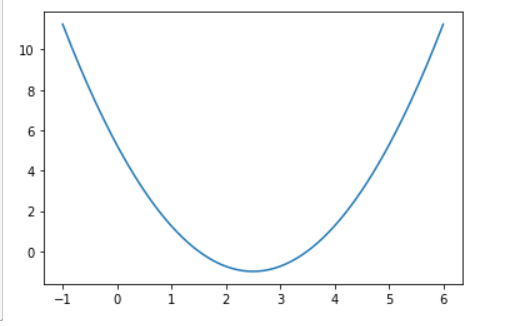def dJ(theta):
return 2*(theta - 2.5)

def J(theta):
try:
return (theta - 2.5)**2-1
except:
return float('inf')


theta= 0.5

theta= 0.9

theta= 1.2200000000000002

theta= 1.4760000000000002

theta= 1.6808

......

theta= 2.4998638870532313

## 画出梯度下降的过程

theta = 0.0
theta_history = [theta]
while True:
last_theta = theta
theta = theta - eta * gradient
theta_history.append(theta)
if(abs(J(theta) - J(last_theta)) < epsilon):
break

plt.plot(plot_x,J(plot_x))
plt.plot(np.array(theta_history),J(np.array(theta_history)),color="r",marker="+")
plt.show()

## 学习率对梯度下降快慢的影响

initial_theta = 0
eta = 0.001
theta_history = []
plot_theta_history()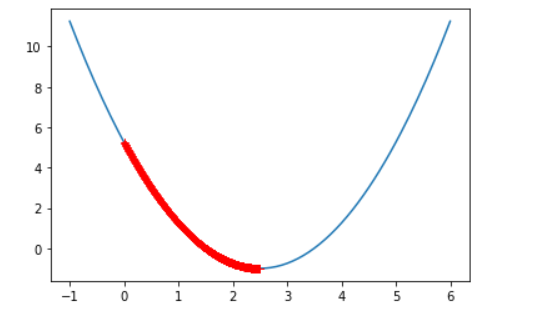initial_theta = 0
eta = 0.8
theta_history = []
plot_theta_history()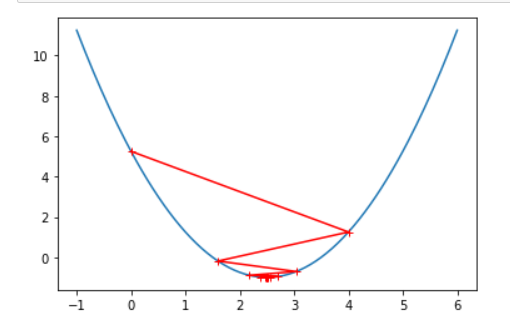initial_theta = 0
eta = 1.1
theta_history = []
plot_theta_history()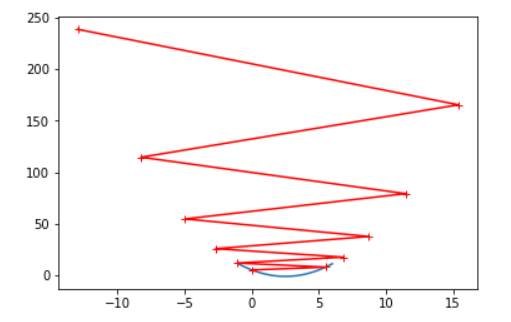# 在线性回归模型中使用梯度下降法

import numpy as np
import matplotlib.pyplot as plt

np.random.seed(666)
x = 2 * np.random.random(size=100)
y = x * 3. + 4. + np.random.normal(size=100)
X = x.reshape(-1, 1)
X.shape
plt.scatter(x, y)
plt.show()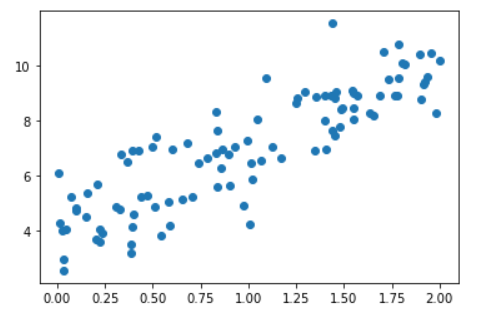def J(theta, X_b, y):
try:
return np.sum((y - X_b.dot(theta))**2) / len(X_b)
except:
return float('inf')

def dJ(theta, X_b, y):
res = np.empty(len(theta))
res = np.sum(X_b.dot(theta) - y)
for i in range(1, len(theta)):
res[i] = (X_b.dot(theta) - y).dot(X_b[:,i])
return res * 2 / len(X_b)

def gradient_descent(X_b, y, initial_theta, eta, n_iters = 1e4, epsilon=1e-8):

theta = initial_theta
cur_iter = 0

while cur_iter < n_iters:
last_theta = theta
theta = theta - eta * gradient
if(abs(J(theta, X_b, y) - J(last_theta, X_b, y)) < epsilon):
break

cur_iter += 1

return theta

X_b = np.hstack([np.ones((len(x), 1)), x.reshape(-1,1)])
initial_theta = np.zeros(X_b.shape)
eta = 0.01
theta = gradient_descent(X_b, y, initial_theta, eta)

# Conclusion（总结）

1.学习率eta取值影响获得最优解的速度

2.学习率eta取值不合适，甚至得不到最优解

3.学习率eta是一个超参数

4.使用梯度下降算法不一定能得到全局最优解，可能是局部最优解

5.可以多次运行，随机化初始点，多次尝试找出最优解

### android make-standalone-toolchain.sh 使用说明-程序员宅基地

#$ANDROID_NDK/build/tools/make-standalone-toolchain.sh --platform=android-24 --install-dir=./android-toolchain --ndk-dir=/Users/musictom/Library/Android/sdk/ndk-bundle/ --use-llvm#$ANDROID_NDK/bui...

### SQLServer 2005 之 海量数据解决方案 分区表-程序员宅基地

http://www.cnblogs.com/yizhu2000/archive/2007/12/13/992901.htmlCsdn Blog在2007年，由于访问量和数据量的大幅度增长，使得我们原有的在.text 0.96版本上修改的代码基本不堪重负。在数据库方面主要表现为，单单文章表，2007年1年的数据已经达到了30G的量（最后的解决方案是对把文章表分为两个表，分别存放文章...

### 290. Word Pattern-程序员宅基地

Given a pattern and a string str, find if str follows the same pattern.Here follow means a full match, such that there is a bijection between a letter in pattern and a non-empty word in str.Exampl...